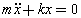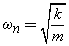# Ship noise and vibration control

Vibration is a complex phenomena happen on ship and machinery space, to understand and have the first sight of vibration assessment or further development, the fundamental of vibration theory is the great article for your pre-learning with such concept.In this topic, i’ll try to make it it as simple as possible to know-how the vibration analysis and modeling solutions in engineering term.

As defined, any motion that repeats itself after an interval of time is called vibration or oscillation.

• 1st Basic modeling objects

A vibratory system, in general, includes a means for storing potential energy – thế năng (spring or elasticity), a means for storing kinetic energy – động năng (mass or inertia), and a means by which energy is gradually lost (damper).

• 2nd Basic vibrating motion

During vibrating, the object will be shifted out the original position and come back like a pendulum as example for a simple harmonic motion ~ Sin Cos motion 🙂 as below:Frequence f = 1/s (Hz), T is the period of one cycle of vibrating motion.

The other exponential, periodic motion will be introduced later.

• 3rd Degree of Freedom – DOF

As complex as vibrating compact system configuration,  an initial step defining how many mathematical particular moving parts that make simple the solutions is very important.DOF – number of coordinates required to express the motion position of systems. (x1,x2,x3)

## Example of Math’ solution for S-DOF systemS-DOF vibration can be analyzed by Newton’s second law of motion, F = m*a.

The analysis can be easily visualized with the aid of a free body diagram,The resulting equation of motion (EOM) is a 2nd-Order Linear Differential Equations (2ndLDE)We will go through this S-DOF case by case as below capture:Case I –  UNDAMPED NATURAL VIBRATION ( c=0, F(t)=0 )

EOM:Resolved above EOM, we found that the Natural Frequency – NF of system:S-DOF UNDAMPED NATURAL RESOLVING

Note that Three solution used to resolve the EOM are called “Harmonic motion”.

NF depends only on the system mass and the spring stiffness (i.e. any damping will not change the natural frequency of a system)

The displacement plot of Case I would appear as:Key note: “To know that every system, has itself Natural vibration”

Case II –  DAMPED NATURAL VIBRATION ( c#0, F(t)=0 )

EOM:Resoled above EOM, found that the solutions fall into one of the following 3 cases depending on the “Damping ratio” values:

S-DOF DAMPED NATURAL RESOLVING

1 – Underdamped Systems (ex: Door closing devices).2 – Critically-Damped Systems3 – Over-Damped SystemsKey note: “Damper always cause reducing the amplitude magnitude upon times”

Case III –  UNDAMPED FORCED VIBRATION ( c=0, F(t)#0 )

By applied a harmony force (simple as machine operation itself) and resolved the EOM we found that the Resonant phenomenal (system con be damaged due to excessive amplitude magnitude)  will happened if the Operation frequency designed too closed to the Natural frequency of system.

Case IV –  DAMPED FORCED VIBRATION ( c#0, F(t)#0 )

With damping devices, the Resonant amplitude can be decreased and the system can be prevented from damaged if any case of failed designate frequency (The frequency of an engine often have the resonant stage at the beginning ).

For resolving the EOM of Case III & IV, see this assumption :

S-DOF FORCED VIBRATION RESOLVING

As found with the benefit of Damper (c), through Damper ratio, the amplitude of motion will be decreased significantly.

Meanwhile, because of the Conservation of Energy – bảo toàn năng lượng, the Force or energy will be transmitted to the ground, deck or their rigid connections.Using vibration isolator (Spring, rubber ect.,) for reducing the Motion amplitude.

Note that the extreme damper doesn’t mean a good idea for vibration control.

Hence, the response of a damped system under the harmonic motion of the Base and the ideas of displacement transmissibility – Td and force transmissibility – Tf  are introduced as below:

For the EOM assumptions:

Isolator and Transmissibility Resolving

Unbalanced Vibration Resolving

The majority of isolators possess damping in varying degrees.

Damping is advantageous when the mounted system is operating at or near its natural frequency because it helps to reduce transmissibility.For example, consider an internal combustion engine mounted on steel springs which possess very little damping . Upon start up of the engine and as the engine RPM increases, the disturbing frequency of the engine will at some point correspond with the natural frequency of the spring-mass system. With light damping the buildup of forces from the engine to the support will be very large or transmissibility will be very high.

If the idle RPM of the engine falls in the range of the natural frequency of the spring-mass system, serious damage may result to the engine or to the support chassis. If, on the other hand, the designer selects an isolator which possesses a higher degree of damping, amplification at resonance would be much less.

It’s not easy for control the vibration from design stage on complex system, so the resonant frequency can happen in occasionally. An introduction about Absorber device is a common solution in this case.2-DOF and Absorber application Resolving